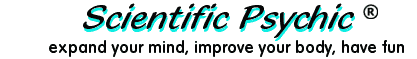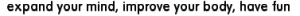Index# Prediction: Your result will be 18

 Think of a number. Add 7 to it. Subtract 2. Subtract your original number. Multiply by 4. Subtract 2.

### Explanation:

This problem gives the illusion of guessing an unknown number, but the unknown has been cancelled mathematically.
( X + 7 - 2 - X ) × 4 - 2 = 18